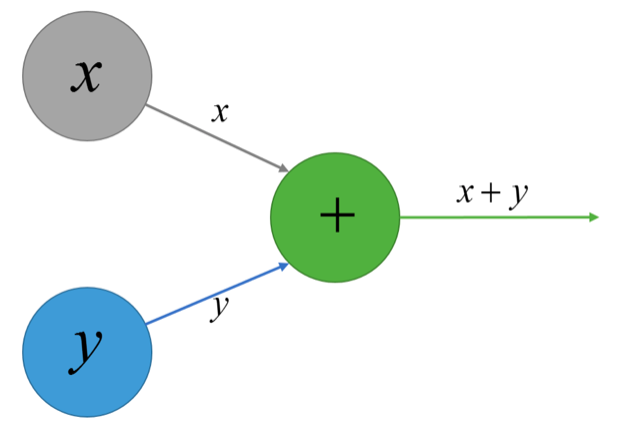Prince Home Stay Follish, Stay Hungry

# 实现一个简易的计算图功能

2019-09-07
Prince### 要实现一个什么样的功能

# 主函数调用
if __name__ == '__main__':

Graph.clear()

# 初始化placeholder
a = PlaceHolder()
b = PlaceHolder()

# 计算图构建
c = a + b
d = b + a
e = c * d + a + b
p = e ** 2

# 计算图喂数据
sess = Session()
print(sess.run([c, d, e], feed_dict={a: 8, b: 2}))
print(sess.run([p], feed_dict={a: 10, b: 20}))


### 1. Tensor定义

TF中所有的计算节点我们都把他们认为是一个Tensor，所谓Tensor是一个虚拟的东西，在构建计算图的时候并不知道他的具体值是多少，只有在喂数据的时候才知道他的具体值是多少，所以我们先定义计算图节点Tensor：

# Tensor抽象类，无法实例化
class Tensor(object):

def __mul__(self, rhs):
return MulTensor(self, rhs)

def __pow__(self, pow):
return PowTensor(self, pow)

def forward(self, feed_dict):
raise NotImplementedError()


# PlaceHolder类，forward直接返回其值
class PlaceHolder(Tensor):

def __init__(self):
Graph.graph[self] = None

def forward(self, feed_dict):
return feed_dict[self]

# 加法Tensor，初始化时保存他的结果是从哪两个值算出来的

def __init__(self, a, b):
Graph.graph[self] = (a, b)

def forward(self, feed_dict):
a, b = Graph.graph[self]
return a.forward(feed_dict) + b.forward(feed_dict)

# 乘法Tensor，初始化时保存他的结果是从哪两个值算出来的
class MulTensor(Tensor):

def __init__(self, a, b):
Graph.graph[self] = (a, b)

def forward(self, feed_dict):
a, b = Graph.graph[self]
return a.forward(feed_dict) * b.forward(feed_dict)

# 一元运算符乘方
class PowTensor(Tensor):

def __init__(self, a, pow=2):
self.pow = pow
Graph.graph[self] = a

def forward(self, feed_dict):
a = Graph.graph[self]
return a.forward(feed_dict) ** self.pow


{
tensor_c: (tensor_a, tensor_b),
tensor_d: (tensor_b, tensor_a)
}


class Graph(object):

graph = {}

def __init__(self):
pass

@staticmethod
def clear():
Graph.graph = {}


class Session(object):

def __init__(self):
pass

def run(self, var_list, feed_dict):
if isinstance(var_list, Tensor):
return var_list.forward(feed_dict)
return [var.forward(feed_dict) for var in var_list]

# tensorflow中也提供这样的函数，不使用操作符
def tensor_sum(a, b):
return a + b

def tensor_mul(a, b):
return a * b


### 还有什么

3. 大量重复计算，细心的读者会发现，在计算sess.run([c, d, e], feed_dict={a: 8, b: 2})的时候，计算e的时候需要用到c的值，而实际上我们通过递归的方法是重复计算了一遍c的值的，性能会有很大的损失，比较好的方法是用记忆化搜索的方式，把所有Tensor的值都存下来，如果再次用到了，不需要重复计算